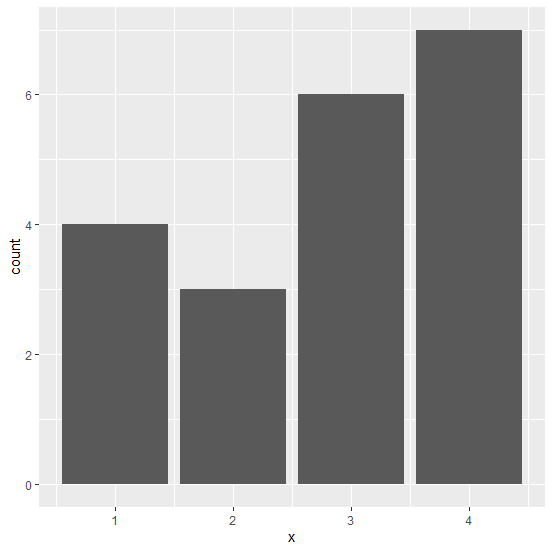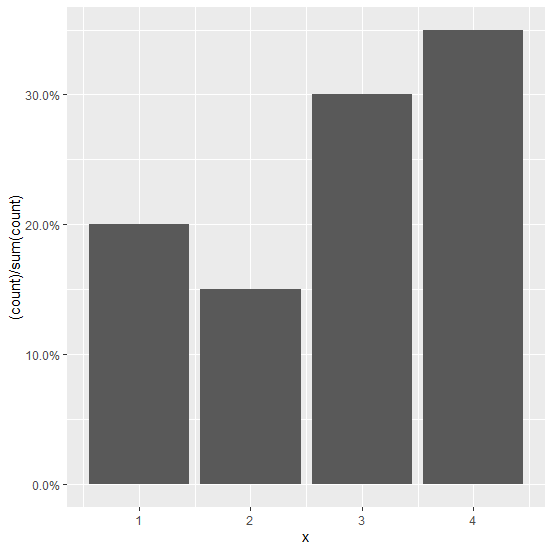# How to create a bar plot using ggplot2 with percentage on Y-axis in R?

Mostly, the bar plot is created with frequency or count on the Y-axis in any way, whether it is manual or by using any software or programming language but sometimes we want to use percentages. It can be done by using scales package in R, that gives us the option labels=percent_format() to change the labels to percentage.

## Example

Consider the below data frame −

Live Demo

> x<-sample(1:4,20,replace=TRUE)
> df<-data.frame(x)
> df

## Output

 x
1 2
2 3
3 3
4 1
5 2
6 4
7 4
8 4
9 2
10 3
11 3
12 4
13 3
14 4
15 4
16 1
17 3
18 1
19 4
20 1

> library(ggplot2)
> ggplot(df,aes(x))+geom_bar()

## OutputLoading scales package and creating the bar plot with percentage on Y-axis −

## Example

> library(scales)
>
ggplot(df,aes(x))+geom_bar(aes(y=(..count..)/sum(..count..)))+scale_y_continuous(labels
=percent_format())

## Output